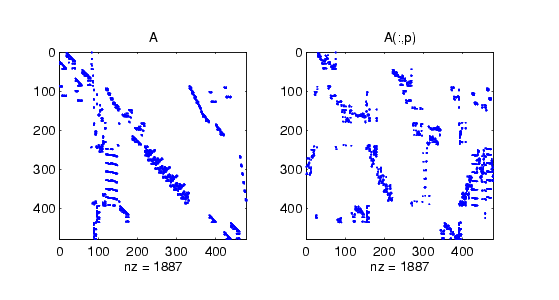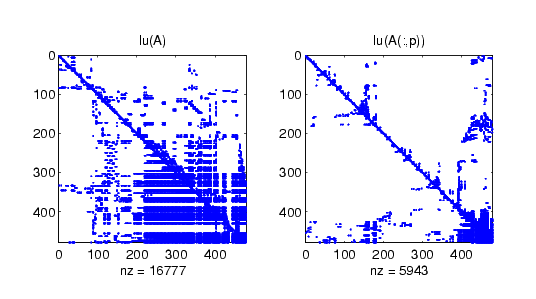MATLAB Function Referencecolamd

Column approximate minimum degree permutation

Syntax

• ```p = colamd(S)
p = colamd(S,knobs)
[p,stats] = colamd(S)
[p,stats] = colamd(S,knobs)
```

Description

```p = colamd(S) ``` returns the column approximate minimum degree permutation vector for the sparse matrix `S`. For a non-symmetric matrix `S`, `S(:,p)` tends to have sparser LU factors than `S`. The Cholesky factorization of `S(:,p)' * S(:,p)` also tends to be sparser than that of `S'*S`.

`knobs` is a two-element vector. If S is `m`-by-`n`, then rows with more than `(knobs(1))*n` entries are ignored. Columns with more than `(knobs(2))*m` entries are removed prior to ordering, and ordered last in the output permutation `p`. If the `knobs` parameter is not present, then `knobs(1)` = `knobs(2) = spparms('wh_frac')`.

`stats` is an optional vector that provides data about the ordering and the validity of the matrix `S`.

 `stats(1)` Number of dense or empty rows ignored by `colamd` `stats(2)` Number of dense or empty columns ignored by `colamd` `stats(3)` Number of garbage collections performed on the internal data structure used by `colamd` (roughly of size `2.2*nnz(S) + 4*m + 7*n` integers) `stats(4)` `0` if the matrix is valid, or `1` if invalid `stats(5)` Rightmost column index that is unsorted or contains duplicate entries, or `0` if no such column exists `stats(6)` Last seen duplicate or out-of-order row index in the column index given by `stats(5)`, or `0` if no such row index exists `stats(7)` Number of duplicate and out-of-order row indices

Although, MATLAB built-in functions generate valid sparse matrices, a user may construct an invalid sparse matrix using the MATLAB C or Fortran APIs and pass it to `colamd`. For this reason, `colamd` verifies that `S` is valid:

• If a row index appears two or more times in the same column, `colamd` ignores the duplicate entries, continues processing, and provides information about the duplicate entries in `stats(4:7)`.
• If row indices in a column are out of order, `colamd` sorts each column of its internal copy of the matrix `S` (but does not repair the input matrix `S`), continues processing, and provides information about the out-of-order entries in `stats(4:7)`.
• If `S` is invalid in any other way, `colamd` cannot continue. It prints an error message, and returns no output arguments (`p` or `stats`) .

The ordering is followed by a column elimination tree post-ordering.

 Note    `colamd` tends to be faster than `colmmd` and tends to return a better ordering.

Examples

The Harwell-Boeing collection of sparse matrices and the MATLAB demos directory include a test matrix `west0479`. It is a matrix of order 479 resulting from a model due to Westerberg of an eight-stage chemical distillation column. The spy plot shows evidence of the eight stages. The `colamd` ordering scrambles this structure.

• ```load west0479
A = west0479;
p = colamd(A);
subplot(1,2,1), spy(A,4), title('A')
subplot(1,2,2), spy(A(:,p),4), title('A(:,p)')
```Comparing the spy plot of the LU factorization of the original matrix with that of the reordered matrix shows that minimum degree reduces the time and storage requirements by better than a factor of 2.8. The nonzero counts are 16777 and 5904, respectively.

• ```spy(lu(A),4)
spy(lu(A(:,p)),4)
```See Also

`colmmd`, `colperm`, `spparms`, `symamd`, `symmmd`, `symrcm`

References

  The authors of the code for `colamd` are Stefan I. Larimore and Timothy A. Davis (`davis@cise.ufl.edu`), University of Florida. The algorithm was developed in collaboration with John Gilbert, Xerox PARC, and Esmond Ng, Oak Ridge National Laboratory. Sparse Matrix Algorithms Research at the University of Florida: http://www.cise.ufl.edu/research/sparse/

© 1994-2005 The MathWorks, Inc.